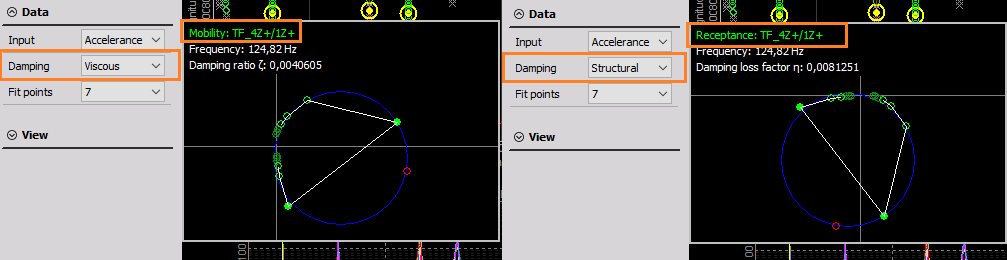# Modal test

Modal test is a process to determine the transfer characteristic of a system. It is most often used to find the natural frequencies of a mechanical structure using hammer or shaker excitations.

• Required hardware: Sirius, Dewe43
• Required software: Dewesoft DSA or SE, PROF and FRF option

## Modal test (introduction)

Modal test is a mathematical module that allows us to calculate the transfer function ($H$) between two signals.

$$H = \frac{\text{Output}}{\text{Input}}$$

Input and Output signals are usually mechanical or electrical nature. Mechanical means exciting structure with hammer or shaker and measure response with an accelerometer. Electrical can be for example input and output voltage of the amplifier in order to get transfer function of the amplifier.

Transfer function ($H$) is a relation between two signals in a complex form (real and imaginary vs frequency).

## Data collection:

Calculations can be done in three ways:

## Setup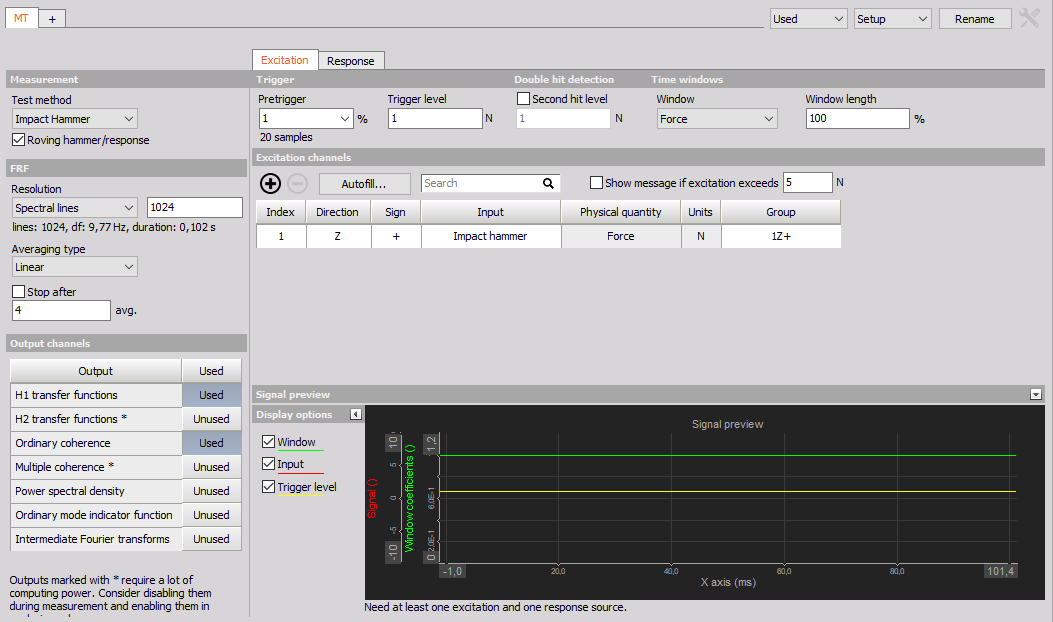### Calculation

Transfer function is calculated via the standard Frequency response function algorithm or FRF for short. It is possible to calculate all excitation/response pair at once or in groups.

#### Test method

• Impact Hammer - In triggered acquisition, blocks of data are taken when trigger level is exceeded.
• Shaker - In Free run blocks of data are taken continuously (one following another with overlap if specified).
• ODS (Operational Deflection  Shapes) -  A single accelerometer is used as means of excitation measurement, others are used for the response. This method is used if excitation by hammer or shaker is not an option.

Use one excitation channel and several response channels or several excitation channels and one response channel in a single measurement.

Do multiple measurements with one or more excitation points to measure one or more responses.

#### FRF estimator

• H1 - The most commonly used estimator is the H1-estimator, which assumes that there is no noise on the input and consequently that all the input measurements are accurate. All noise is assumed to be on the output.
• H2 - Alternatively, the H2 estimator can be used. This assumes that there is no noise on the output and consequently that all the output measurements are accurate. Noise is assumed to be only on input.

### FRF resolution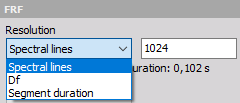The resolution of results can be detemined with selecting:

• Spectral lines - The number of lines for FFT (block size equals two times lines).
• Df [Hz] - delta frequency, frequency resolution of the results.
• Segment duration [s] - duration of the block segment.

### FRF (frequency response function)

• Averaging type - Select the averaging type from Linear, Peak, Exponent

• Stop after - Stop after the set number of averages

### Stop after

Select the check-box to enable Averaging and input the maximum number of measurements. This will enable you to take the predefined number of measurements and average them for a combined result. If the number of averages is not reached, the result will be calculated from the completed measurements. After the number is reached, you will not be able to do additional measurements for that group.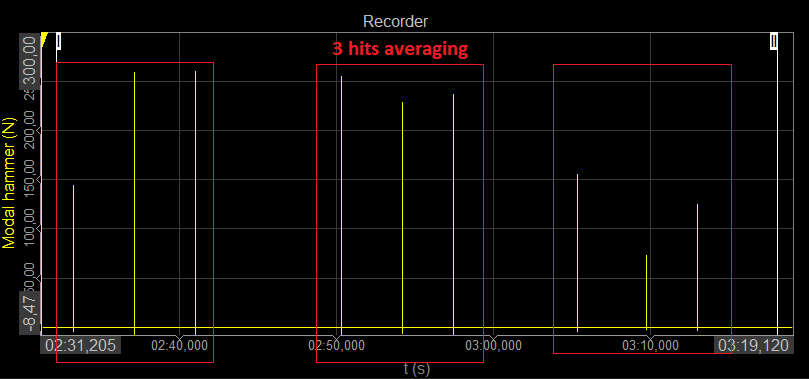### Output channels

• Ordinary mode indicator function - Mode Indicator Function is a function ranging from zero to one and shows where resonances are.
• Ordinary coherence - is a real value between zero and one. If the value is one then response power is caused totally by input power. A value less then one indicates that measured response power is greater than that from input power (noise…).
• Multiple coherence - used in MIMO calculation. If the value is one then response power is caused totally by input power. A value less then one indicates that measured response power is greater than that from input power (noise…).
• PSD - Power Spectral Density is the measure of signal’s power content over frequency.
• Intermediate Fourier transform - FFTs of excitation and response channels during the measurement.

### Impact hammer

#### Impact hammer triggering

• Pretrigger: defines how much data will be taken before a trigger is reached (in per cent of block size). If this is set to zero we will probably miss some data on the beginning.
• Trigger level: trigger level defines the level that trigger channel must reach in order to recognize a hit
• Second hit level: after the actual hit, if the second hit level is reached, double hit is detected

#### Time windows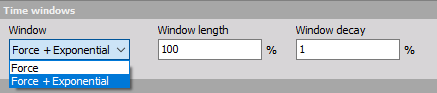• Force: this will aplly a rectanular window in excitation channels (in selected %) and rectangular window (100%) to response channels
• Force + Exponential: this will apply an exponential window on excitation and response channels

#### Double hit detection

Enable or disable second hit level detection with the check box and enter the desired value in the field below. The signal will be searched for peaks and if there is more than one peak which is higher than double hit detection level you will get a warning that double hit happened. You can check it on excitation spectrum. Then you can reject this measurement or continue.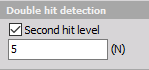### Shaker

#### Excitation source

• External - Shaker is controlled by an external signal

• Burst - define the pretrigger, trigger level and windowing
• Continuous - define the FFT window and overlap
• Dewesoft AO - shaker is controlled (open loop) by Dewesoft’s Function generato (Analog Out)

• Continuous random - define the FFT window and overlap
• Burst random - define the excitation duration (in % of the segment duration), pretrigger and trigger level
• Sine sweep - define the waveform, start, stop frequency and sweep time

All the seting from Function generator can be defined directly in Modal test module.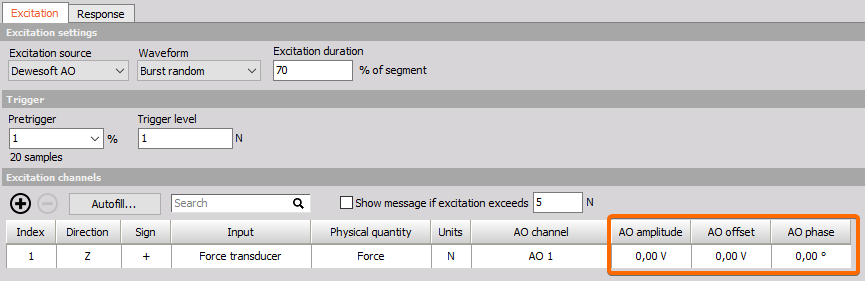### Operational deflection shapes (ODS)

In ODS mode settings are similar to the Shaker method, except that we can’t use the Function generator and we are limited to one Reference “excitation” channel.

In ODS, one of the accelerometers is selected as the reference channel and other are selected as response channels.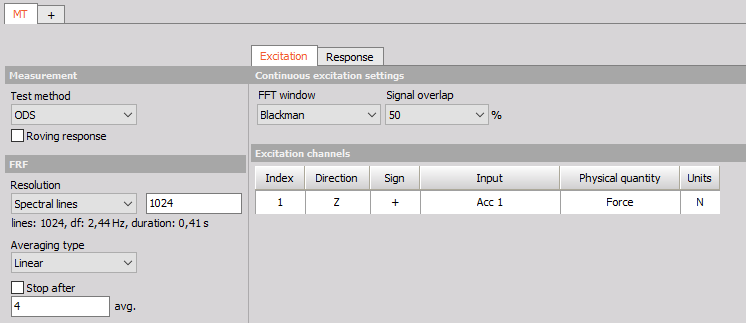### Excitation and response channel settings

Show message if excitation exceeds - This is just a safety that we don’t damage our structure. When the response acceleration from our system is above the limit analogue out and measurement are stopped.

Excitation and response configurations

• Index - index of measured point, connects the tranfer function with a dedicated points in the geometry
• Direction - defines the direction (x, y, z) in which we measure certain point (related to animation of the geometry).
• Sign - defines the orientation of the sensor (+, -)
• Input - Input channel that is physically connected (force or an acceleration sensor).
• Physical input and unit - input and unit of the input channel
• Group - Define the measurement group when using Roving hammer/response. All the sensors defined in the same group, wil be measured at the same time.
• AO channel - Analog Output channel which is used for driving shaker.
• AO amplitude - amplitude of analog out signal
• AO offset - offset of analog out signal
• AO phase - phase of analog out signal

### Signal preview

Directly in Modal test module we offer a user to be able to see the preview if excitation and response channels.

With this preview the user can correctly set the trigger level and windowing.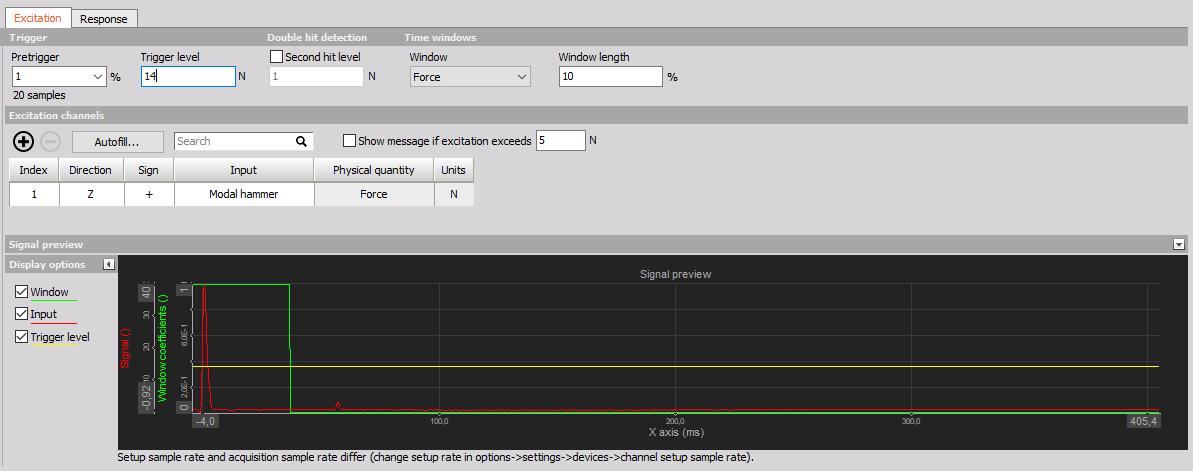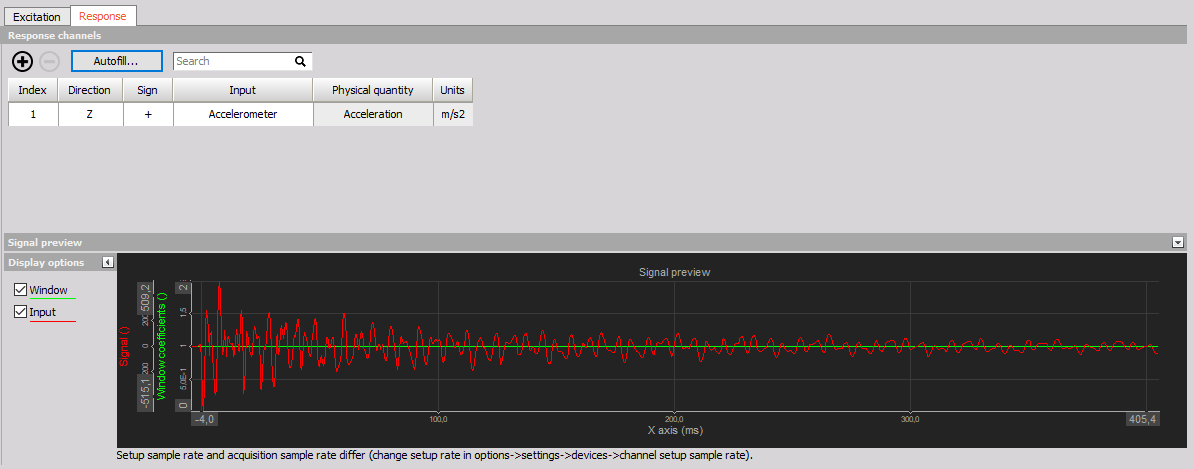### Autofill setup

NOTE: If you wish to use Autofill (for response channels) for triaxial sensors you must connect the response channels (Triaxial accelerometers) in a manner of: 1st sensor X, Y, Z, 2nd sensor X, Y, Z, and so on.

Input channels settings

AI start node input channel - Select the channel that will measure the first node of the structure Triaxial sensors (only for response ch.) - If multi-axial sensors are used, check the box to define the used channels under Geometry settings / Directions

Geometry settings

• Start node index - Define the starting point node of the structure
• Node index increment - Set the increment by which the nodes will advance
• Direction(s) - Select the direction(s) of the measurement (if Triaxial response  you can select multiple)
• Sign - Define if the excitation will be positive or negative regarding the Direction.
• Sensors in group - Define the number of sensors in one rowing group
• Roving count - Define the number of positions, how many moves of the sensor group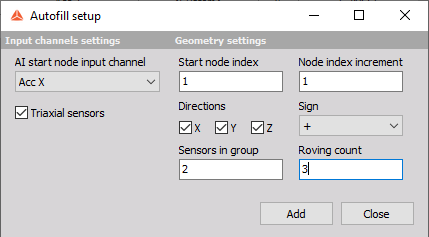### Outputs channels

• Transfer function (H1 or H2 estimator)
• Amplitude - signal amplitude.
• Phase - signal phase.
• Real - the real part of a signal.
• Imaginary - the imaginary part of a signal.
• Ordinary mode indicator function - Mode Indicator Function is a function ranging from zero to one and shows where resonances are.
• Ordinary coherence - is a real value between zero and one. If the value is one then response power is caused totally by input power. A value less then one indicates that measured response power is greater than that from input power (noise…).
• Multiple coherence - used in MIMO calculation. If the value is one then response power is caused totally by input power. A value less then one indicates that measured response power is greater than that from input power (noise…).
• PSD - Power Spectral Density is the measure of signal’s power content over frequency.
• Intermediate Fourier transform - FFTs of excitation and response channels during the measurement.

Common channels

• Info - information about which point is currently measured.
• Ave Count - a number of collected averages.
• Reject last - this is a channel which can be used with visual control to reject last sample (overload of channels, double hit…).
• Next point - this is a channel which can be used with visual control to go to the next point when grouping is used.

## Measure

Modal test module automatically creates pre-defined measurement display.

In predefined display you are able to see the progress table, FFTs of excitation and response channels, transfer functions, coherences and geometry.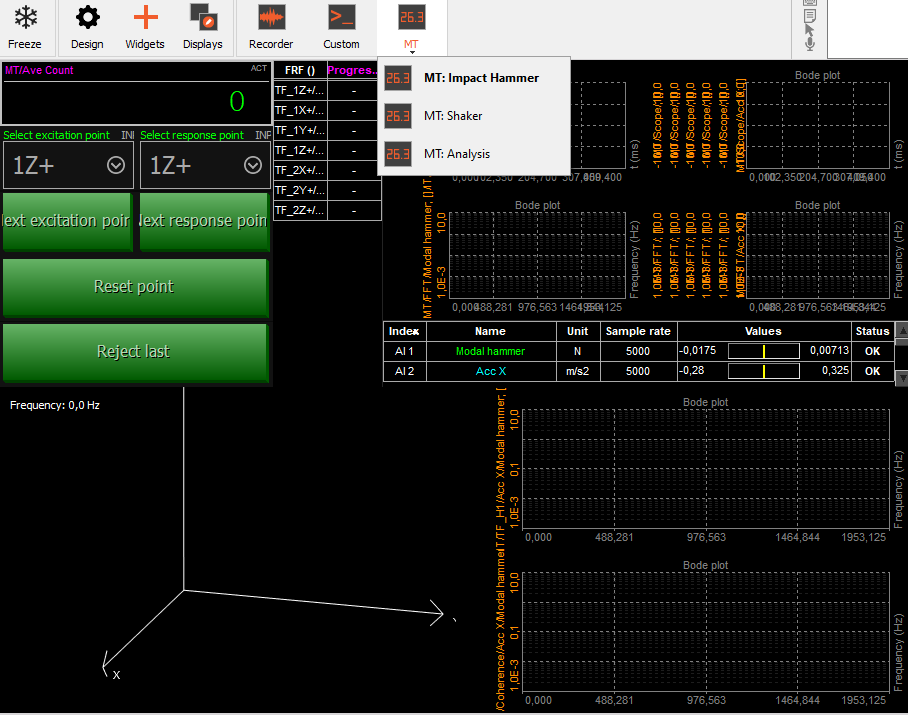### Visualize behaviour of the strucutre

How to visualize animation of the structure with FRF Geometry visual control see Modal geometry.

### Progress table

In the Pogress table all the transfer function are were defined in Modal test module are listed. When a tranfer functions of each group are calculated you will see a green indicator. Until the groups is measured, it will remain red.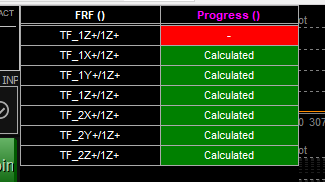### Circle fit (exact damping estimation)

This is a visual control for basic modal analysis. You can use the yellow cursor in a 2D graph and set it to the frequency content of interest. Circle fit will find the nearest peak (resonance) and fit the circle into that data set. Then it will calculate the damping factor and more exact frequency (between line resolution of FFT).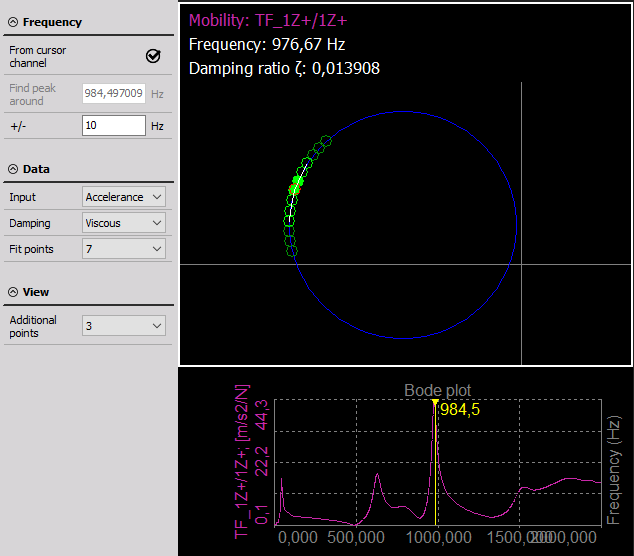• From cursor channel - the yellow cursor on the 2D graph is taken for frequency point determination.
• Manual - you can manually enter frequency point for circle fit procedure.
• Peak search (manual mode) - an area in which we will search for peak for circle fit.
• Neighbour count - a number of neighbours taken into account when doing the circle fit.

Dewesoft Modal circle visual control lets the user to chose between the structural and viscous damping model and calculate either damping loss factor or damping ratio from receptance or mobility, after first integrating or differentiating the measured FRF to get the correct form.

If we assume a structural damping model, the receptance forms a perfect circle on the Nyquist plot. The contribution of other modes can be neglected.

For the viscous damping model, we used the Nyquist plot of Mobility which likewise forms a circle-like curve around the resonance.